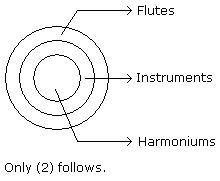# Verbal Reasoning - Syllogism - Discussion

Discussion Forum : Syllogism - Syllogism 1 (Q.No. 2)
Directions to Solve

In each of the following questions two statements are given and these statements are followed by two conclusions numbered (1) and (2). You have to take the given two statements to be true even if they seem to be at variance from commonly known facts. Read the conclusions and then decide which of the given conclusions logically follows from the two given statements, disregarding commonly known facts.

• (A) If only (1) conclusion follows
• (B) If only (2) conclusion follows
• (C) If either (1) or (2) follows
• (D) If neither (1) nor (2) follows and
• (E) If both (1) and (2) follow.

2.

Statements: All the harmoniums are instruments. All the instruments are flutes.

Conclusions:

1. All the flutes are instruments.
2. All the harmoniums are flutes.

Only (1) conclusion follows
Only (2) conclusion follows
Either (1) or (2) follows
Neither (1) nor (2) follows
Both (1) and (2) follow
Explanation:Discussion:
70 comments Page 1 of 7.

Yangdol said:   4 months ago
Let's analyse the given statements and draw conclusions based on them:.

Given Statements:.

1. All the harmoniums are instruments.
(This can be represented as: All Harmoniums (H) are Instruments (I)).

2. All the instruments are flutes.
(This can be represented as: All Instruments (I) are Flutes (F)).

Conclusions:.

1. All the flutes are instruments.
(This can be represented as: All Flutes (F) are Instruments (I)).

2. All the harmoniums are flutes.
(This can be represented as: All Harmoniums (H) are Flutes (F)).

Let's evaluate each conclusion:.
All the flutes are instruments.
This conclusion can be derived from the second statement (All instruments are flutes). So, it follows logically. (True).
All the harmoniums are flutes.

This conclusion cannot be derived from the given statements. We know that all harmoniums are instruments, and all instruments are flutes, but this doesn't necessarily mean that all harmoniums are flutes. (False).

So, the correct option is:

A) Only (1) conclusion follows.

Explanation:

The first conclusion (All the flutes are instruments) follows logically from the given statements because it is directly stated in the second premise.

However, the second conclusion (All the harmoniums are flutes) does not necessarily follow from the given statements. Just because all harmoniums are instruments and all instruments are flutes doesn't imply that all harmoniums are flutes. There could be other instruments besides flutes that harmoniums might fall under.

Hence, this conclusion is not valid.
(3)

Thanusri said:   5 months ago
Option B is correct.

Only 2 conclusion follows is the right answer.
(3)

Soumya said:   1 year ago
All harmonium are instruments.

But not all instruments are flutes.

So, in my opinion, the answer is A.
(15)

Deb Nc said:   2 years ago
Both the statement has instrument. That means All harmoniums and flutes are instrument. So, the biggest circle should be instrument.

E is not the answer because conclusion 1 is the reversed statement 2. It is not valid.

To get the conclusion, we will omit the same word from 1 & 2 statement. Then we will relate the remaining words from 2 statements.
(2)

Shivam said:   3 years ago

Prakash said:   4 years ago
Instead of following this diagrammatic representation, if we follow the syllogism rules basis it would be easier and we can solve it in less than minute.
(4)

Hanuman said:   4 years ago
@Ravinder, I learned Raval Notations, this is simple, fast and accurate. By Raval notations HH -I II -F , since common term (I) multiplies, so HH - F follows. Can Raval Notations can solve possibility type questions too, last time I was not able to crack IBPS Exam by two marks and I feel with knowledge of Raval Notations, I will have around 4 minutes extra

Abhishek said:   4 years ago
Conclusion and statement are not equal. If both are same. There is no concept of conclusion. Remember one think conclusion means we get some thing from statement. So conclusion is becomes statement. What you learn from conclusion. That's why option 1 is wrong.

Ayush said:   4 years ago
Set of instruments and set of flute can also overlap so answer might be (d).
(1)

Ravinder said:   4 years ago
@Hanuman.

Statements (both premises and conclusions) are represented as follows: Statement Notation
a) All S are P, SS-P
b) Some S are P, S-P
c) Some S are not P, S / PP
d) No S is P, SS / PP

RULES:

1) Conclusions are reached by connecting Notations. Two notations can be linked only through common linking terms. When the common linking term multiplies (becomes double from single), divides (becomes single from double) or remains double then the conclusion is arrived between terminal terms.

2) If both statements linked are having " signs, resulting conclusion carries " sign
3) Whenever statements having " and / signs are linked, resulting conclusion carries / sign.
4) Statement having / sign cannot be linked with another statement having / sign to derive any conclusion.

For this question:
By Raval notations HH -I II -F so HH - F follows.